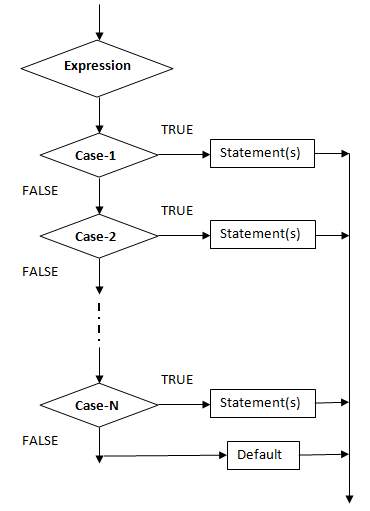## switch-case statement

View

This statement is used to develop menu- driven programs. Menu-driven programs are those in which user is provided with the options to select based on his choice. For Example-

2.      Subtract two numbers
3.      Multiply two numbers
4.      Divide two numbers
5.      Exit
3
Enter First number
10
Enter Second number
5
Product = 50

2.      Subtract two numbers
3.      Multiply two numbers
4.      Divide two numbers
5.      Exit
1
Enter First number
10
Enter Second number
5
Total = 15

2.      Subtract two numbers
3.      Multiply two numbers
4.      Divide two numbers
5.      Exit
5

Syntax-

switch ( expression)
{
case 1:             statement(s);
break;
case 2:             statement(s);
break;
case 3:             statement(s);
break;
.
.
.
case N:            statement(s);
break;
default:           statement(s);
}

FlowchartExample-1

#include < stdio.h >
#include < conio.h >
#include < process.h >
void main ( )
{
// Variable Declaration
int choice;
float a, b, c ;
clrscr ( );
// Processing and Output
printf ( “\n 1. Add two numbers”);
printf ( “\n 2. Subtract two numbers”);
printf ( “\n 3. Multiply two numbers”);
printf ( “\n 4. Divide two numbers”);
printf ( “\n 5. Exit”);
printf ( “\n Enter your choice (1-5)”);
scanf(“ %d “, &choice );
switch ( choice )
{
case 1:printf ( “\n Enter First Number \n “);
scanf(“ %f “, &a );
printf ( “\n Enter Second Number \n “);
scanf(“ %f “, &b );
c = a + b;
printf ( “\n Total = %f“, c);
break;
case 2:printf ( “\n Enter First Number \n “);
scanf(“ %f “, &a );
printf ( “\n Enter Second Number \n “);
scanf(“ %f “, &b );
c = a - b;
printf ( “\n Difference = %f“, c);
break;
case 3:printf ( “\n Enter First Number \n “);
scanf(“ %f “, &a );
printf ( “\n Enter Second Number \n “);
scanf(“ %f “, &b );
c = a * b;
printf ( “\n Product = %f“, c);
break;
case 4:printf ( “\n Enter First Number \n “);
scanf(“ %f “, &a );
printf ( “\n Enter Second Number \n “);
scanf(“ %f “, &b );
c = a / b;
printf ( “\n Division = %f“, c);
break;
case 5:exit(0);
default:printf ( “\n Wrong Choice \n “);
}
getch ( );
}